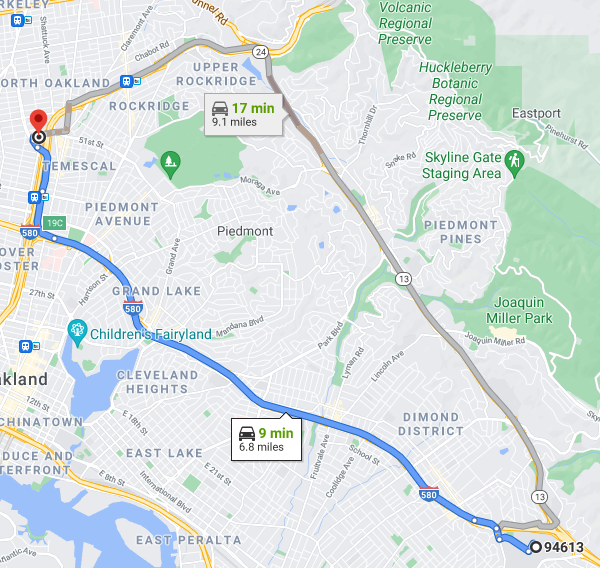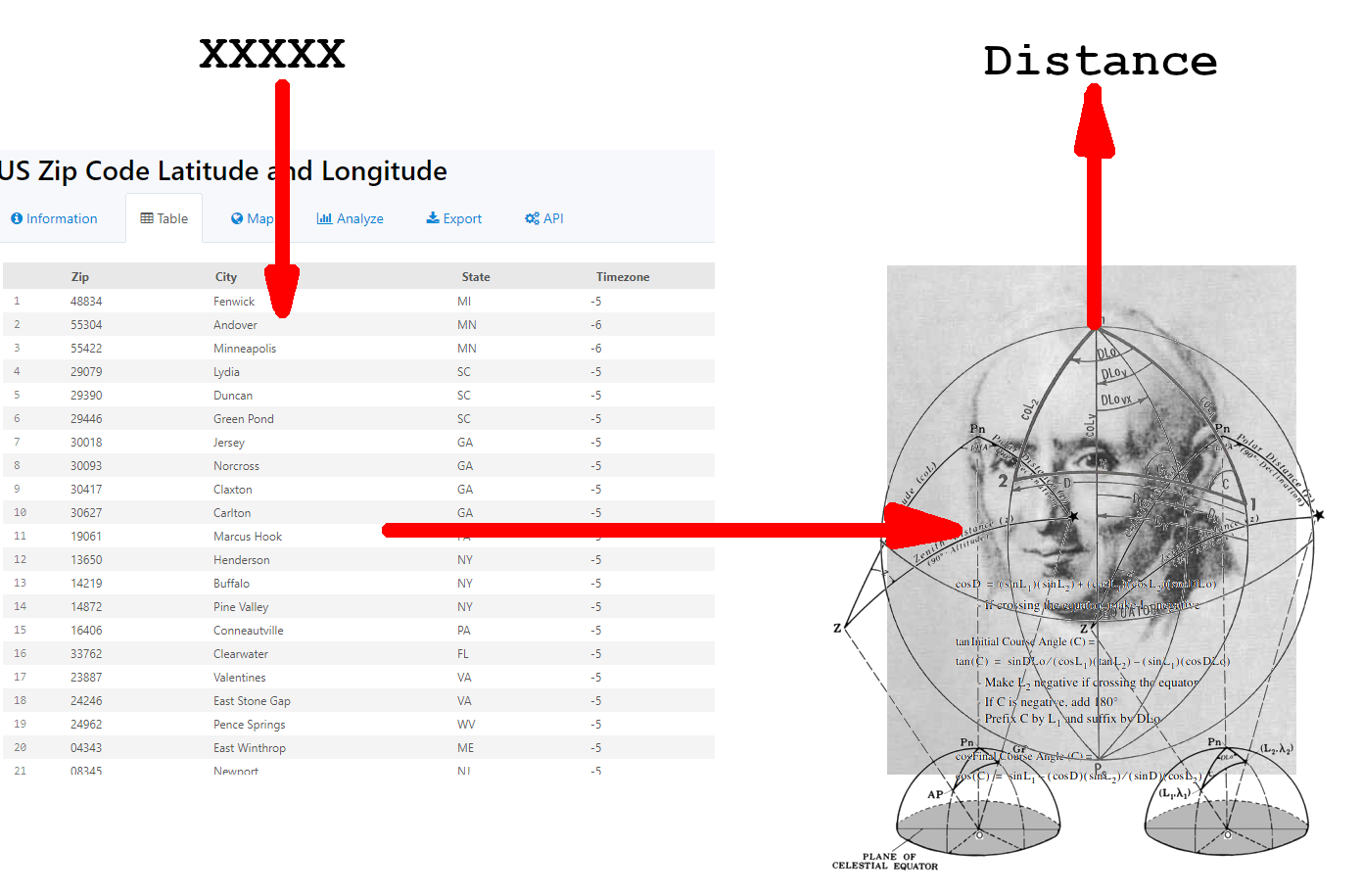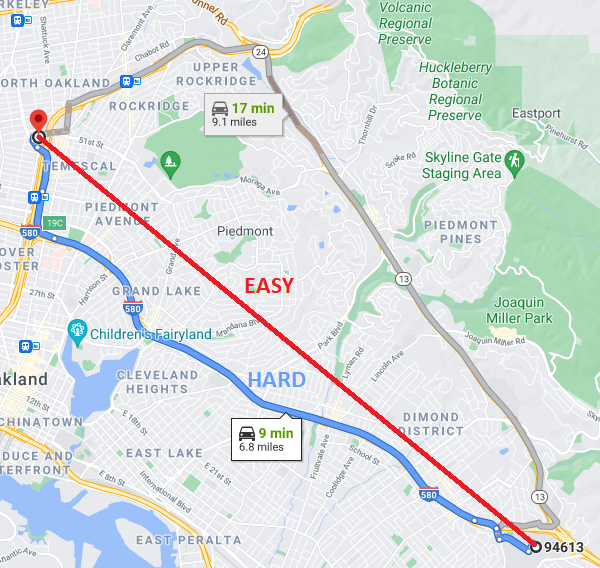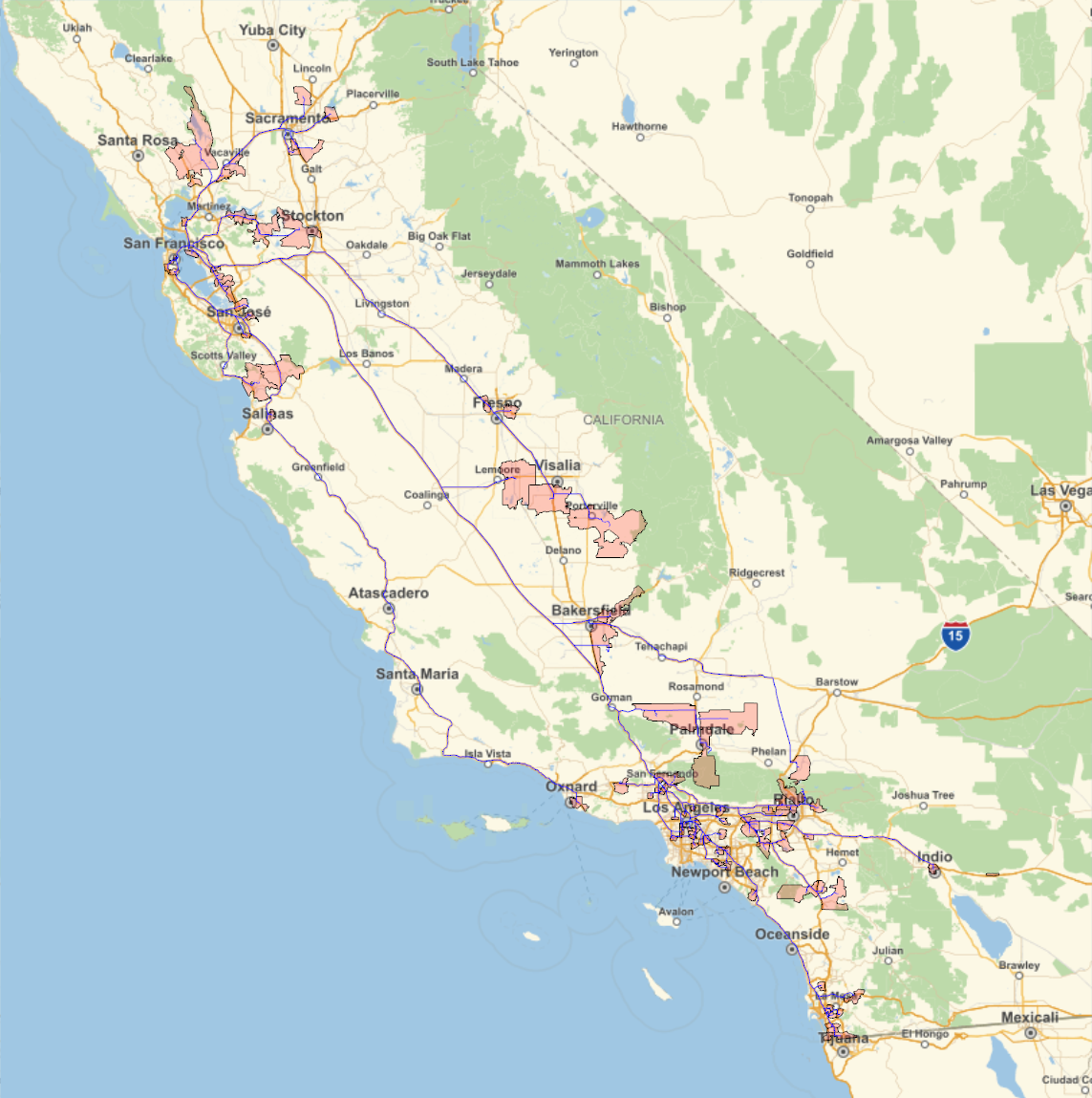# Blog

## How Far is That By Car?

### 20 May 2021

All of this code is on Github, so check it out, if you can!

My wife, Jackie, is a medical student at UCSF, but this week she visited me! So far, we've had a ton of fun in Norfolk, and we have some great plans for the weekend.

Without fail, Jackie brings me new interesting problems every time she comes here. Right now, she's working on determining the distances from patients' homes to the hospital. For anonymity, only patients' zip codes are used. Essentially, the problem can be stated like this:

How can you find the distance between a given zipcode and a given hospital?

She wants to take a list of zipcodes (in an excel doc or CSV file) and turn it into a list of zipcodes with added columns for distances to certain hospitals. With one or two zipcodes, it's easy. Just plug them into google maps. For example, here is the distance between a randomly selected zipcode (94613) and Benioff Children's Hospital in Oakland.If you have a hundred (or a thousand) zipcodes, though, it might be more useful to do this with code. I thought it was an interesting problem so I decided to give it a try.

Having read Bowditch on deployment while mortally bored, I had a few initial instincts about how to tackle this problem. I needed a way to convert zip codes to lat/longs, and then a way to find the distance between the lat/longs and the hospital.

For the zip code conversions, I could use a table like this with list matching . Once I had the latitude and longitude, I could use this with the hard-coded lat/long of the hospital to find the distance.

Finding the distance between points on the Earth's surface is a fairly complex problem. When the points are close together, you can use a flat earth approximation. This treats the earth as a flat grid of latitude and longitude. While a degree of latitude is always around 60 nautical miles (69 statute miles), the length of a degree of longitude changes with latitude, because longitudinal lines are closer together at the poles. This length is about $$\frac{\pi}{180}r_e\cos\phi$$, where $$r_e$$ is the Earth's radius and $$\phi$$ is the latitude. Unfortunately, some of the zipcodes were fairly far apart, so this approximation would not be accurate. Thankfully, Bowditch figured this all out in Chapter 12 of the American Practical Navigator. If we assume that the Earth is a perfect sphere (it's not, but this gives about 1% accuracy), we can use a Great Circle Sailing formula to find the distance:$d=r_e \mathrm{arccos}\left(\sin\phi_1\sin\phi_2+\\\cos\phi_1\cos\phi_2\cos(\Delta\lambda)\right)$ $$d$$ is the distance, $$r_e$$ is Earth's mean radius, $$\phi_1$$ and $$\phi_2$$ are the latitudes, and $$\Delta\lambda$$ is the absolute change in longitude. For less math (but more work), one could use the Sight Reduction Tables published by the NGA. There are a lot of great online tools for doing this as well, like Great Circle Mapper.

After a little reading, I had a pretty good idea of how my program would work. This was what I had in my mind's eye:Then Jackie dropped the bomb: she didn't just want the great circle distances from these zip codes, she wanted the driving distances. Bowditch didn't own a car, so he never thought about it, but this is a harder problem which requires a lot more specifics about the road structure of the US.Fortunately, Google has been working on this problem for years, and they have a lot of tools available to amateur programmers. They have several different APIs for accomplishing different tasks with Google Maps. Python has a good client for all these APIs which I decided to use, since Python is an easy, fun programming language to experiment with, and I didn't need the awesome performance of C. Installing this was as easy as:

In order to use these APIs, Google requires you to generate an API key. I was a little wary of this at first, because I don't love having my queries tracked, and it seemed like an easy way to trick me into spending money, but the pricing model was fairly transparent, and convinced me that this would be free unless I called the API 40000 times this month (which I didn't plan to).

I ended up using the Distance Matrix API, which finds the distances between addresses. Another benefit of using Google is that it natively supports zipcodes, so I didn't need to convert them to lat/longs (using, for example, the table above). Finding the distance between two points was as easy as:

This would return a weird,complicated dict object like this:

To find the actual distance just required a little hunting through this object:

This is the distance in meters; divide by 1609.34 to get the distance in miles. $$\frac{10982}{1609.34} \approx 6.82\text{ miles}$$

Which is the same as what we found on google maps when we did the search manually. That's all there is to this API!

Jackie already had the list of zipcodes in a vertical CSV file (she is using RStudio for her data analysis). For the purpose of demonstration, I have generated a similar file, but made of the 100 most populous zips in California. Manipulating this file just required a little knowledge of the Python CSV module.

I defined a function called distance_to_hospital, which found the distance from a zip to the Oakland and San Francisco hospitals. Then I iterated this function over the list of zipcodes, and finally I saved the results as a new CSV called output.

As a sort of 'dessert', I used Mathematica to visualize the paths from each zip code. Mathematica has a few really fun features, like the ability to plot out a zip code's polygonal shape, and the travel directions from place to place. Since it's proprietary software, I prefer not to post a lot of work in it, but it can help make some pretty sweet visuals, like the following driving map.No clinical data was used in this demonstration. Zip codes were selected based on population, not patient location.